# Differentiation in Calculus Chapter Exam

Exam Instructions:

Choose your answers to the questions and click 'Next' to see the next set of questions. You can skip questions if you would like and come back to them later with the yellow "Go To First Skipped Question" button. When you have completed the practice exam, a green submit button will appear. Click it to see your results. Good luck!

### Page 1

#### Question 1 1. According to the mean value theorem, at how many points will the instantaneous rate of change equal the average rate of change between x = 1 and x = 3 on the following graph?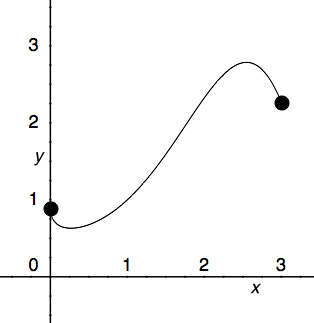#### Question 2 2. Which point on the following graph satisfies the mean value theorem between x = 0 and x = 3?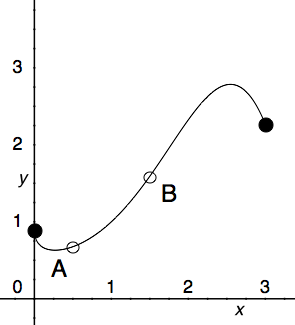#### Question 3 3. What is the approximate instantaneous rate of change at point C?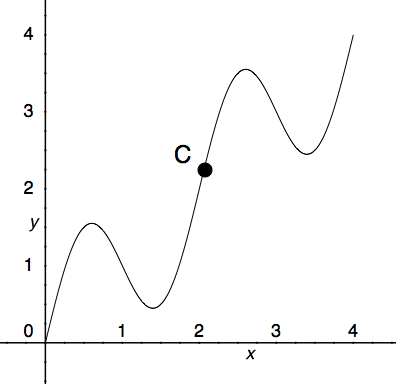#### Question 4 4. Find the derivative df/dt, given the function f(t).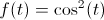#### Question 5 5. Evaluate.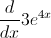### Page 2

#### Question 6 6. Find the derivative of f(x).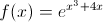#### Question 7 7. Evaluate the derivative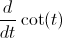#### Question 8 8. Given the derivative of a function (ƒ′(x)), which of the following could be ƒ(x)?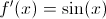#### Question 9 9. Find the derivative of f(x).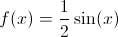### Page 3

#### Question 13 13. Find the second derivative of the following function.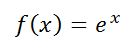#### Question 14 14. Find the second derivative.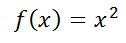#### Question 15 15. Find the second derivative.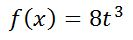### Page 4

#### Question 16 16. Consider the graph below. Between x=C and x=-C, for any value of C, will there be a point where the instantaneous rate of change is 0?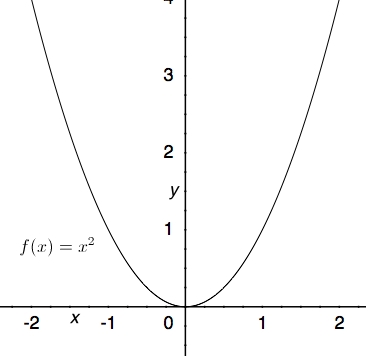#### Question 18 18. Consider the function f(x) given below. According to Rolle's theorem, between x=0 and x=pi, for how many values of x is the instantaneous rate of change equal to 0?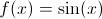#### Question 19 19. Find the derivative of p(t).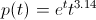#### Question 20 20. Find the derivative of f(x).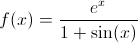### Page 5

#### Question 21 21. Evaluate.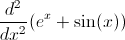#### Question 22 22. How many smaller functions does this function have?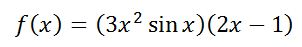#### Question 23 23. Take the derivative of the following function.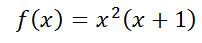#### Question 24 24. Does this function need the use of the product rule?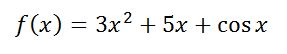#### Question 25 25. Find the derivative of f(x).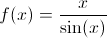### Page 6

#### Question 26 26. Find f'(t) given the function f(t):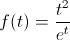#### Question 27 27. Find y'.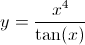#### Question 29 29. Find f'(x) for the following function.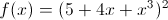#### Question 30 30. Find the derivative of f(x).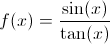#### Differentiation in Calculus Chapter Exam Instructions

Choose your answers to the questions and click 'Next' to see the next set of questions. You can skip questions if you would like and come back to them later with the yellow "Go To First Skipped Question" button. When you have completed the practice exam, a green submit button will appear. Click it to see your results. Good luck!

Support